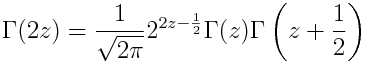# Every factorial is a power

The previous post mentioned that 24! ≈ 1024 and 25! ≈ 1025.

For every n, there is some base b such that n! = bn. For example, 30! ≈ 1230.

It’s easy to find b :What’s interesting is that b is very nearly a linear function of n.In hindsight it’s clear that this should be the case—it follows easily from Stirling’s approximation—but I didn’t anticipate this before I plotted it.

Now fix n and find b such that n! = bn. Since the relationship between n and b(n) is nearly linear, this suggestswhich is true. It follows from the multiplication identity for the gamma function:Let z = n + 1/2 so that the left side is (2n)!. On the right side, Γ(z + 1/2) = n! and Γ(z) is not too different from n!. The rest of the right side is 22n/√π.

So our observation that b(n) is nearly linear gave us a hint of Gauss’s multiplication formula.

 Numerically you would probably evaluate this function by calling a routine that computes log Γ(n + 1) directly without computing Γ(n + 1) first. This avoids overflow for large n.

This is why mathematical libraries will have not only gamma functions but also loggamma functions. The latter seems redundant, but it’s not.

## 4 thoughts on “Every factorial is a power”

1. Michael Albert

The approximation you note could also be seen by observing that $C(2n, n) = \frac{(2n)!}{(n!)^2}$ on the one hand, and (as the central binomial) is also at least $2^{2n}/n$ (in fact more like $2^{2n}/\sqrt{n}$ of course by central limit stuff.)

2. Martin Cohen

The basic approximation I use is
n! ~ (n/e)^n so ln(n!) ~ n(ln(n)-1).
The basic inequality is
(n/e)^n < n! < (n/e)^{n+1}.
When considering convergence of series using the n-th root test,
(n!)^{1/n} ~ n/e so
((an)!)^{1/n} = (((an)!)^{1/(an)})^a ~(an/e)^a = a^an^a/e^a.

3. From above:
24! ≈ 10^24
25! ≈ 10^25
but:
25! = 24! * 25
10^24*25 != 10^24*10

Your approximately equal must be quite approximate.

4. The approximations are fairly accurate on a log scale. This post was a follow on to a post on estimating the order of magnitude of factorials.